# Coherent Detection of DSB-SC Waves

## Coherent Detection of DSB-SC Waves

The coherent detection of the DSB-SC signal is shown in fig.1 .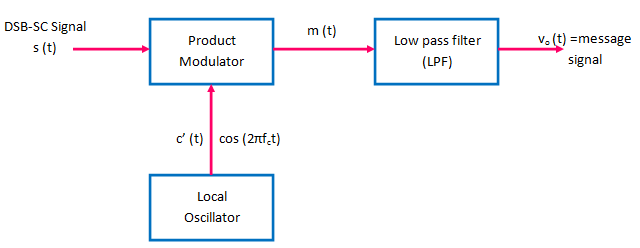Fig 1: Coherent Detection of DSB-SC modulated wave

The DSB-SC wave s(t) is applied to a product modulator in which it is multiplied with the locally generated carrier cos (2πfct) .

We assume that this locally generated carrier is exactly coherent or synchronized in both frequency and phase with the original carrier wave c(t) used to generate the DSB-SC wave .

This method of detection is therefore called as coherent detection or synchronous detection .

The output of the product modulator is applied to the low pass filter (LPF) which eliminates all the unwanted frequency components and produces the message signal .

## Analysis of Coherent Detection

Let the output of the local oscillator be given by :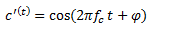Thus its amplitude is 1, frequency is fand the phase difference is arbitrary equal to φ .

This phase difference has been measured with respect to the original carrier c(t) at the DSB-SC generator .

Therefore, the output of the product modulator is given by :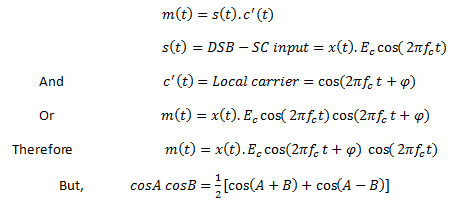Hence,Thus,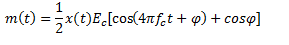Therefore,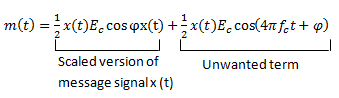The above equation shows that the output of product modulator i.e. m(t) consists of two terms. The first one represents the message signal x(t) with an amplitude of (1/2) Eccosφ . Hence, this is the wanted term. The second term is an unwanted one .

Signal m(t) is then passed through a low pass filter, which allows only the first term to pass through and will reject the second term .

Therefore, the filter output is given by :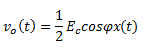Thus, output voltage of the coherent demodulator is proportional to  the message signal x(t) if the phase  error cosφ is constant .

### Effect of Phase Error on the Demodulated Output

Let us consider the expression for the output of coherent detector is given by :In this expression, φ represents the phase error and the amplitude of the demodulated output is maximum and equal to (1/2) Ewhen φ = 0o and the amplitude is zero when  φ = 90.

This effect is called as the quadrature null effect of the coherent detector .

Here, quadrature term represents the phase difference of  90o.

In other words, the phase error attenuates the demodulator output .

In practice, the phase error varies randomly with time due to the random variations taking place in the communication channel . Hence, cosφ will vary randomly and the detector output also will vary in a random manner.

This is undesirable . hence, circuitry must be provided in the detector to keep the locally generated carrier c'(t) in perfect synchronism, in both frequency and phase, with the original carrier c(t) .